matplotlib.pyplot.axis¶

matplotlib.pyplot.axis(*v, **kwargs)[source]

Convenience method to get or set axis properties.

Calling with no arguments:

>>> axis()

returns the current axes limits [xmin, xmax, ymin, ymax].:

>>> axis(v)

sets the min and max of the x and y axes, with v = [xmin, xmax, ymin, ymax].:

>>> axis('off')

turns off the axis lines and labels.:

>>> axis('equal')

changes limits of x or y axis so that equal increments of x and y have the same length; a circle is circular.:

>>> axis('scaled')

achieves the same result by changing the dimensions of the plot box instead of the axis data limits.:

>>> axis('tight')

changes x and y axis limits such that all data is shown. If all data is already shown, it will move it to the center of the figure without modifying (xmax - xmin) or (ymax - ymin). Note this is slightly different than in MATLAB.:

>>> axis('image')

is 'scaled' with the axis limits equal to the data limits.:

>>> axis('auto')

and:

>>> axis('normal')

are deprecated. They restore default behavior; axis limits are automatically scaled to make the data fit comfortably within the plot box.

if len(*v)==0, you can pass in xmin, xmax, ymin, ymax as kwargs selectively to alter just those limits without changing the others.

>>> axis('square')

changes the limit ranges (xmax-xmin) and (ymax-ymin) of the x and y axes to be the same, and have the same scaling, resulting in a square plot.

The xmin, xmax, ymin, ymax tuple is returned

xlim(), ylim()
For setting the x- and y-limits individually.

Examples using matplotlib.pyplot.axis¶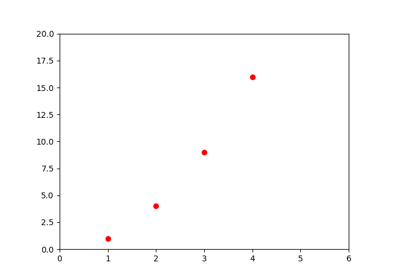Pyplot Formatstr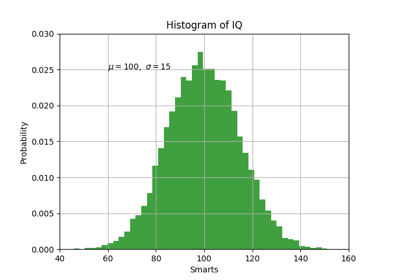Pyplot Text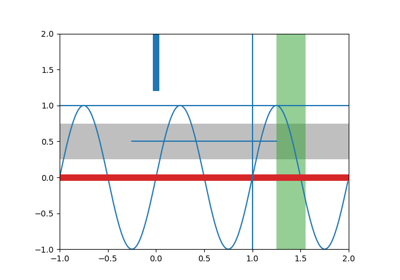axhspan Demo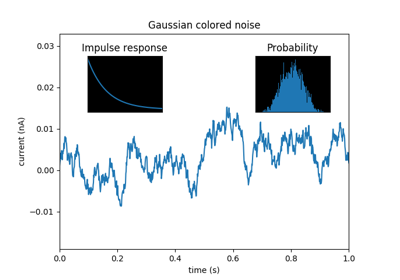Axes Demo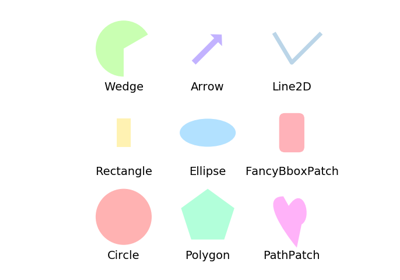Reference for matplotlib artists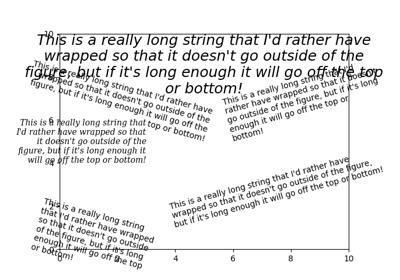Auto-wrapping text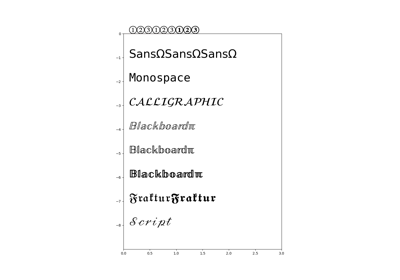STIX Fonts DemoFont Table TTF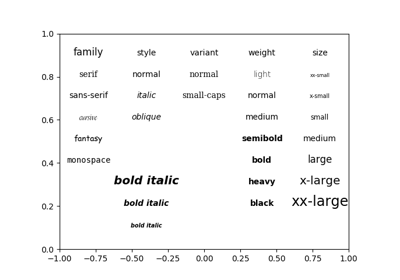Fonts demo (kwargs)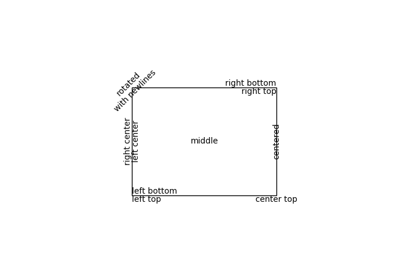Precise text layout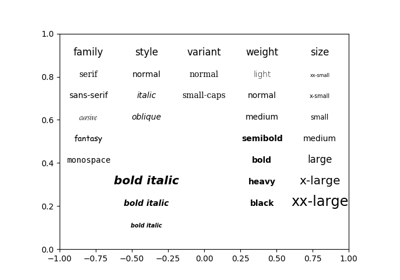Fonts demo (object-oriented style)Interactive functions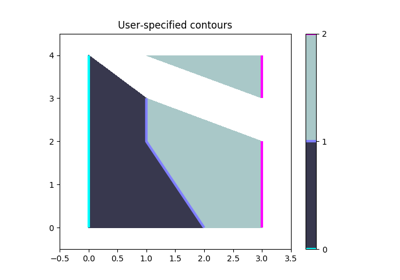Manual ContourCursor Demo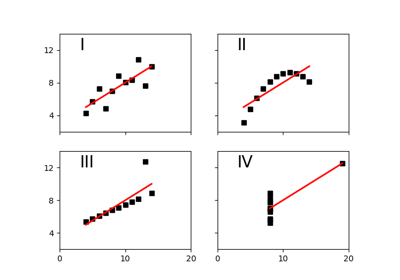Anscombe's Quartet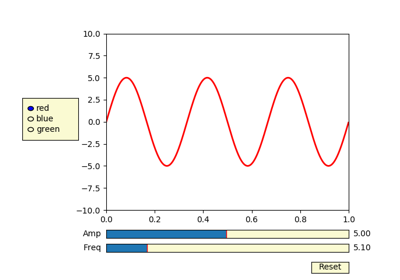Slider Demo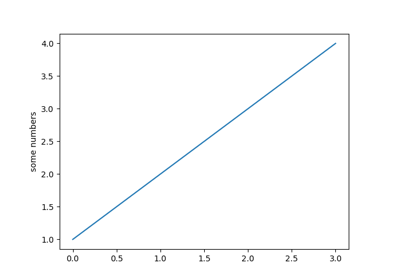Pyplot tutorial